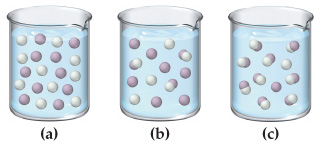# Problem: The following three diagrams represent three different solutions of the binary acid HA. Water molecules have been omitted for clarity and hydronium ions (H3O+) are represented by hydrogen ions (H+). Rank the acids in order of decreasing acid strength.

###### FREE Expert Solution

Recall that:

• Strong acid dissociates completely — HA → H+ + A-
• Weak acid dissociates partially — HA H+ + A-
• The weaker the acid, the lesser the dissociation.

We are given the following figure:

79% (93 ratings)###### Problem Details

The following three diagrams represent three different solutions of the binary acid HA. Water molecules have been omitted for clarity and hydronium ions (H3O+) are represented by hydrogen ions (H+). Rank the acids in order of decreasing acid strength.